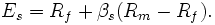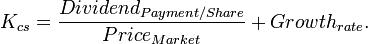### cost of capital

cost of capital

The cost of capital is a term used in the field of financial investment to refer to the cost of a company's funds (both debt and equity), or, from an investor's point of view "the shareholder's required return on a portfolio company's existing securities". It is used to evaluate new projects of a company as it is the minimum return that investors expect for providing capital to the company, thus setting a benchmark that a new project has to meet.

## Summary

For an investment to be worthwhile, the expected return on capital must be greater than the cost of capital. The cost of capital is the rate of return that capital could be expected to earn in an alternative investment of equivalent risk. If a project is of similar risk to a company's average business activities it is reasonable to use the company's average cost of capital as a basis for the evaluation. A company's securities typically include both debt and equity, one must therefore calculate both the cost of debt and the cost of equity to determine a company's cost of capital. However, a rate of return larger than the cost of capital is usually required.
The cost of debt is relatively simple to calculate, as it is composed of the rate of interest paid. In practice, the interest-rate paid by the company can be modeled as the risk-free rate plus a risk component (risk premium), which itself incorporates a probable rate of default (and amount of recovery given default). For companies with similar risk or credit ratings, the interest rate is largely exogenous (not linked to thto the cost of debt, the cost of equity is broadly defined as the risk-weighted projected return required by investors, where the return is largely unknown. The cost of equity is therefore inferred by comparing the investment to other investments (comparable) with similar risk profiles to determine the "market" cost of equity. It is commonly equated using the CAPM formula (below), although articles such as Stulz 1995 question the validity of using a local CAPM versus an international CAPM- also considering whether markets are fully integrated or segmented (if fully integrated, there would be no need for a local CAPM).
Once cost of debt and cost of equity have been determined, their blend, the weighted-average cost of capital (WACC), can be calculated. This WACC can then be used as a discount rate for a project's projected cash flows.

## Cost of debt

When companies borrow funds from outside or take debt from financial institutions or other resources the interest paid on that amount is called cost of debt. The cost of debt is computed by taking the rate on a risk free bond whose duration matches the term structure of the corporate debt, then adding a default premium. This default premium will rise as the amount of debt increases (since, all other things being equal, the risk rises as the amount of debt rises). Since in most cases debt expense is a deductible expense, the cost of debt is computed as an after tax cost to make it comparable with the cost of equity (earnings are after-tax as well). Thus, for profitable firms, debt is discounted by the tax rate. The formula can be written as (Rf + credit risk rate)(1-T), where T is the corporate tax rate and Rf is the risk free rate.
The yield to maturity can be used as an approximation of the cost of debt.

## Cost of equity

Cost of equity = Risk free rate of return + Premium expected for risk
Cost of equity = Risk free rate of return + Beta x (market rate of return- risk free rate of return) where Beta= sensitivity to movements in the relevant market$E_s = R_f + \beta_s(R_m - R_f).\,$
Where:
Es
The expected return for a security
Rf
The expected risk-free return in that market (government bond yield)
βs
The sensitivity to market risk for the security
RM
The historical return of the stock market/ equity market
(RM-Rf)
The risk premium of market assets over risk free assets.
The risk free rate is taken from the lowest yielding bonds in the particular market, such as government bonds.
An alternative to the estimation of the required return by the CAPM as above, is the use of the Fama–French three-factor model.

### Expected return

The expected return (or required rate of return for investors) can be calculated with the "dividend capitalization model", which is$K_{cs} = \frac{Dividend_{Payment/Share}} {Price_{Market}} + Growth_{rate}.\,$

The models state that investors will expect a return that is the risk-free return plus the security's sensitivity to market risk times the market risk premium.
The risk premium varies over time and place, but in some developed countries during the twentieth century it has averaged around 5%. The equity market real capital gain return has been about the same as annual real GDP growth. The capital gains on the Dow Jones Industrial Average have been 1.6% per year over the period 1910-2005.  The dividends have increased the total "real" return on average equity to the double, about 3.2%.
The sensitivity to market risk (β) is unique for each firm and depends on everything from management to its business and capital structure. This value cannot be known "ex ante" (beforehand), but can be estimated from ex post (past) returns and past experience with similar firms.

#### Cost of retained earnings/cost of internal equity

Note that retained earnings are a component of equity, and therefore the cost of retained earnings (internal equity) is equal to the cost of equity as explained above. Dividends (earnings that are paid to investors and not retained) are a component of the return on capital to equity holders, and influence the cost of capital through that mechanism.

## Weighted average cost of capital

The Weighted Average Cost of Capital (WACC) is used in finance to measure a firm's cost of capital.
The total capital for a firm is the value of its equity (for a firm without outstanding warrants and options, this is the same as the company's market capitalization) plus the cost of its debt (the cost of debt should be continually updated as the cost of debt changes as a result of interest rate changes). Notice that the "equity" in the debt to equity ratio is the market value of all equity, not the shareholders' equity on the balance sheet.To calculate the firm’s weighted cost of capital, we must first calculate the costs of the individual financing sources: Cost of Debt, Cost of Preference Capital and Cost of Equity Cap.
Calculation of WACC is an iterative procedure which requires estimation of the fair market value of equity capital.

## Capital structure

Because of tax advantages on debt issuance, it will be cheaper to issue debt rather than new equity (this is only true for profitable firms, tax breaks are available only to profitable firms). At some point, however, the cost of issuing new debt will be greater than the cost of issuing new equity. This is because adding debt increases the default risk - and thus the interest rate that the company must pay in order to borrow money. By utilizing too much debt in its capital structure, this increased default risk can also drive up the costs for other sources (such as retained earnings and preferred stock) as well. Management must identify the "optimal mix" of financing – the capital structure where the cost of capital is minimized so that the firm's value can be maximized.
The Thomson Financial league tables show that global debt issuance exceeds equity issuance with a 90 to 10 margin.
The structure of capital should be determined considering the weighted average cost of capital.

## Modigliani-Miller theorem

If there were no tax advantages for issuing debt, and equity could be freely issued, Miller and Modigliani showed that, under certain assumptions, the value of a leveraged firm and the value of an unleveraged firm should be the same.